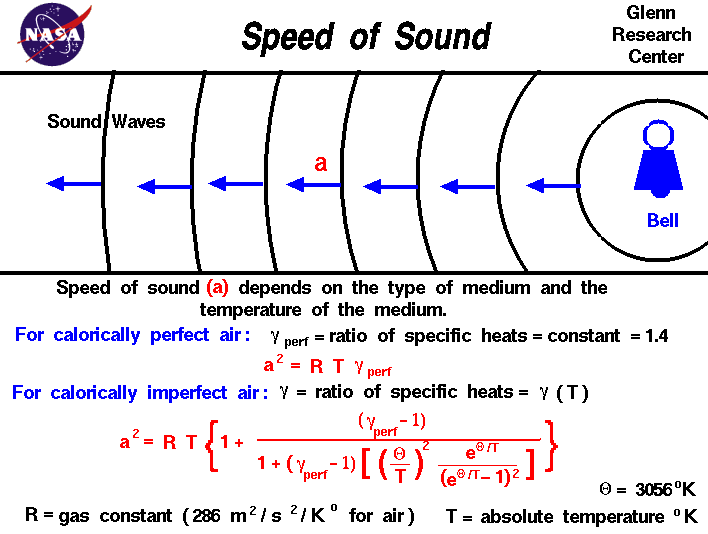+ Text Only Site
+ Non-Flash Version
+ Contact GlennAir is a gas, and a very important property of any gas is the speed of sound through the gas. Why are we interested in the speed of sound? The speed of "sound" is actually the speed of transmission of a small disturbance through a medium. Sound itself is a sensation created in the human brain in response to sensory inputs from the inner ear. (We won't comment on the old "tree falling in a forest" discussion!) Disturbances are transmitted through a gas as a result of collisions between the randomly moving molecules in the gas. The transmission of a small disturbance through a gas is an isentropic process. The conditions in the gas are the same before and after the disturbance passes through. Because the speed of transmission depends on molecular collisions, the speed of sound depends on the state of the gas. The speed of sound is a constant within a given gas and the value of the constant depends on the type of gas (air, pure oxygen, carbon dioxide, etc.) and the temperature of the gas. For hypersonic flows, the high temperature of the gas generates real gas effects that can alter the speed of sound. An analysis based on conservation of mass and momentum shows that the square of the speed of sound a^2 is equal to the the gas constant R times the temperature T times the ratio of. specific heats gamma a^2 = R * T * gamma Notice that the temperature must be specified on an absolute scale (Kelvin or Rankine). The dependence on the type of gas is included in the gas constant R. which equals the universal gas constant divided by the molecular weight of the gas, and on the ratio of specific heats. If the specific heat capacity of a gas is a constant value, the gas is said to be calorically perfect and if the specific heat capacity changes, the gas is said to be calorically imperfect. At subsonic and low supersonic Mach numbers, air is calorically perfect. But under low hypersonic conditions, air is calorically imperfect. Derived flow variables, like the speed of sound and the isentropic flow relations are slightly different for a calorically imperfect gas than the conditions predicted for a calorically perfect gas because some of the energy of the flow excites the vibrational modes of the diatomic molecules of nitrogen and oxygen in the air. The equation given above was derived for a calorically perfect gas. If we include the effects of caloric imperfection, some additional terms are added to the equation. For the calorically imperfect case, mathematical models based on a simple harmonic vibrator have been developed. The details of the analysis were given by Eggars in NACA Report 959. A synopsis of the report is included in NACA Report 1135. To a first order approximation, the equation for the speed of sound for a calorically imperfect gas is given by: a^2 = R * T * {1 + (gamma - 1) / ( 1 + (gamma-1) * [(theta/T)^2 * e^(theta/T) /(e^(theta/T) -1)^2]) } where theta is a thermal constant equal to 3056 degrees Kelvin, gamma is the calorically perfect value of the ratio of specific heats, and T is the static temperature. The speed of sound in air depends on the type of gas and the temperature of the gas. On Earth, the atmosphere is composed of mostly diatomic nitrogen and oxygen, and the temperature depends on the altitude in a rather complex way. Scientists and engineers have created a mathematical model of the atmosphere to help them account for the changing effects of temperature with altitude. We have created an atmospheric calculator to let you study the variation of sound speed with altitude. Here's another Java program to calculate speed of sound and Mach number for different altitudes and speed. You can use this calculator to determine the Mach number of an aircraft at a given speed and altitude. This page shows an interactive Java applet which calculates the speed of sound and the mach number for an input velocity and altitude. To change input values, click on the input box (black on white), backspace over the input value, type in your new value. Then hit the red COMPUTE button to send your new value to the program. You will see the output boxes (yellow on black) change value. You can use either English or Metric units and you can input either the Mach number or the speed by using the menu buttons. Just click on the menu button and click on your selection. There is a sleek version of this program for experienced users who do not need these instructions. You can also download your own copy of this program to run off-line by clicking on this button: Activities: Guided Tours Navigation ..Beginner's Guide Home Page+ Inspector General Hotline + Equal Employment Opportunity Data Posted Pursuant to the No Fear Act + Budgets, Strategic Plans and Accountability Reports + Freedom of Information Act + The President's Management Agenda + NASA Privacy Statement, Disclaimer, and Accessibility CertificationEditor: Tom Benson NASA Official: Tom Benson Last Updated: May 07 2021 + Contact Glenn